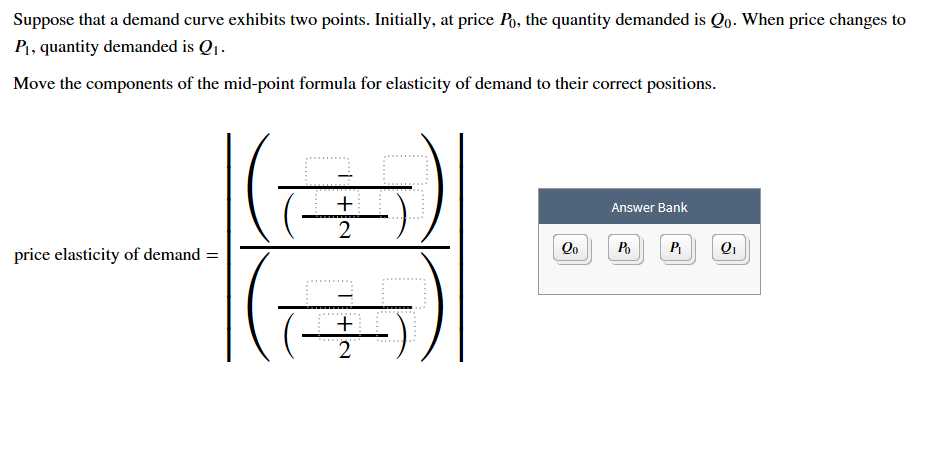Suppose that a demand curve exhibits two points. Initially, at price Po, the quantity demanded is Qo. When price changes toPI, quantity demanded is QMove the components of the mid-point formula for elasticity of demand to their correct positionsAnswer Bank2QoPoQ1price elasticity of demand =2

Questionhelp_outlineImage TranscriptioncloseSuppose that a demand curve exhibits two points. Initially, at price Po, the quantity demanded is Qo. When price changes to PI, quantity demanded is Q Move the components of the mid-point formula for elasticity of demand to their correct positions Answer Bank 2 Qo Po Q1 price elasticity of demand = 2 fullscreen
Step 1

Price elasticity of demand is the percentage changes in quan...

Want to see the full answer?

See Solution

Want to see this answer and more?

Our solutions are written by experts, many with advanced degrees, and available 24/7

See Solution
Tagged in